Friday, 13 March 2015 07:22

## How to Apply Conditional Formatting to a Data Range in C#

Written by  support iceblue
Rate this item
(1 Vote)

Conditional formatting in Microsoft Excel has a number of presets that enables users to apply predefined formatting such as colors, icons and data bars, to a range of cells based on the value of the cell or the value of a formula. Conditional formatting usually reveals the data trends or highlights the data that meets one or more formulas.

In this article, I made an example to explain how these conditional formatting types can be achieved programmatically using Spire.XLS in C#. First of all, let's see the worksheet that contains a group of data in selected range as below, we’d like see which cells’ value is bigger than 800. In order to quickly figure out similar things like this, we can create a conditional formatting rule by formula: “If the value is bigger than 800, color the cell with Red” to highlight the qualified cells.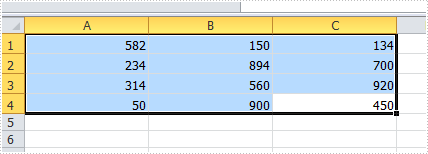#### Code Snippet for Creating Conditional Formatting Rules:

Step 1: Create a worksheet and insert data to cell range from A1 to C4.

```Workbook workbook = new Workbook();
Worksheet sheet = workbook.Worksheets;

sheet.Range["A1"].NumberValue = 582;
sheet.Range["A2"].NumberValue = 234;
sheet.Range["A3"].NumberValue = 314;
sheet.Range["A4"].NumberValue = 50;
sheet.Range["B1"].NumberValue = 150;
sheet.Range["B2"].NumberValue = 894;
sheet.Range["B3"].NumberValue = 560;
sheet.Range["B4"].NumberValue = 900;
sheet.Range["C1"].NumberValue = 134;
sheet.Range["C2"].NumberValue = 700;
sheet.Range["C3"].NumberValue = 920;
sheet.Range["C4"].NumberValue = 450;
sheet.AllocatedRange.RowHeight = 15;
sheet.AllocatedRange.ColumnWidth = 17;
```

Step 2: Create one conditional formatting rule to highlight cells that are greater than 800, and another rule that enables to highlight cells lesser than 300. In our program, the rule is represented by formula. As is shown in the code below, we firstly initialize a new instance of ConditionalFormatWrapper class and apply the format1 to selected cell range. Then define the format1 by setting the related properties. The FirstFormula and Operater property allow us to find out which cells are greater than 800; the Color property enables to color the cells we find. Repeat this method to create format2 to get the cells under 300 highlighted.

```ConditionalFormatWrapper format1 = sheet.AllocatedRange.ConditionalFormats.AddCondition();
format1.FormatType = ConditionalFormatType.CellValue;
format1.FirstFormula = "800";
format1.Operator = ComparisonOperatorType.Greater;
format1.FontColor = Color.Red;
format1.BackColor = Color.LightSalmon;

format2.FormatType = ConditionalFormatType.CellValue;
format2.FirstFormula = "300";
format2.Operator = ComparisonOperatorType.Less;
format2.FontColor = Color.Green;
format2.BackColor = Color.LightBlue;
```

Step 3: Save and launch the file

```workbook.SaveToFile("sample.xlsx", ExcelVersion.Version2010);
System.Diagnostics.Process.Start("sample.xlsx");
```

Result:

The cells with value bigger than 800 and smaller than 300, have been highlighted with defined text color and background color.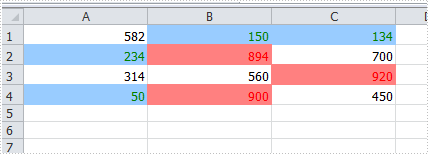#### Apply the Other Three Conditional Formatting Types:

Spire.XLS also supports applying some other conditional formatting types which were predefined in MS Excel. Use the following code snippets to get more formatting effects.

Apply Data Bars:

```ConditionalFormatWrapper format = sheet.AllocatedRange.ConditionalFormats.AddCondition();
format.FormatType = ConditionalFormatType.DataBar;
```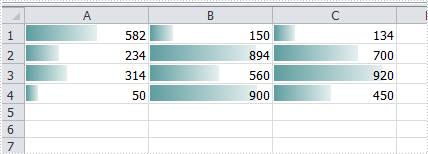Apply Icon Sets:

```ConditionalFormatWrapper format = sheet.AllocatedRange.ConditionalFormats.AddCondition();
format.FormatType = ConditionalFormatType.IconSet;
```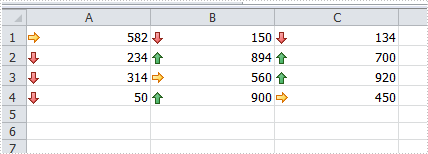Apply Color Scales:

```ConditionalFormatWrapper format = sheet.AllocatedRange.ConditionalFormats.AddCondition();
format.FormatType = ConditionalFormatType.ColorScale;
```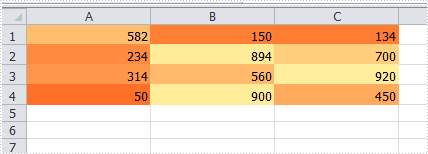Full Code:

```Workbook workbook = new Workbook();
Worksheet sheet = workbook.Worksheets;
sheet.Range["A1"].NumberValue = 582;
sheet.Range["A2"].NumberValue = 234;
sheet.Range["A3"].NumberValue = 314;
sheet.Range["A4"].NumberValue = 50;
sheet.Range["B1"].NumberValue = 150;
sheet.Range["B2"].NumberValue = 894;
sheet.Range["B3"].NumberValue = 560;
sheet.Range["B4"].NumberValue = 900;
sheet.Range["C1"].NumberValue = 134;
sheet.Range["C2"].NumberValue = 700;
sheet.Range["C3"].NumberValue = 920;
sheet.Range["C4"].NumberValue = 450;
sheet.AllocatedRange.RowHeight = 15;
sheet.AllocatedRange.ColumnWidth = 17;

//create conditional formatting rule
format1.FormatType = ConditionalFormatType.CellValue;
format1.FirstFormula = "800";
format1.Operator = ComparisonOperatorType.Greater;
format1.FontColor = Color.Red;
format1.BackColor = Color.LightSalmon;

format2.FormatType = ConditionalFormatType.CellValue;
format2.FirstFormula = "300";
format2.Operator = ComparisonOperatorType.Less;
format2.FontColor = Color.Green;
format2.BackColor = Color.LightBlue;

//format.FormatType = ConditionalFormatType.DataBar;

//format.FormatType = ConditionalFormatType.IconSet;

//format.FormatType = ConditionalFormatType.ColorScale;

workbook.SaveToFile("sample.xlsx", ExcelVersion.Version2010);
System.Diagnostics.Process.Start("sample.xlsx");
```